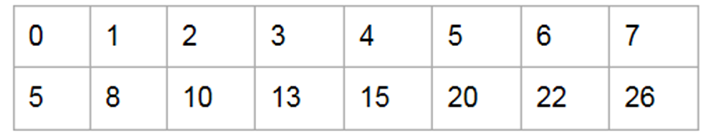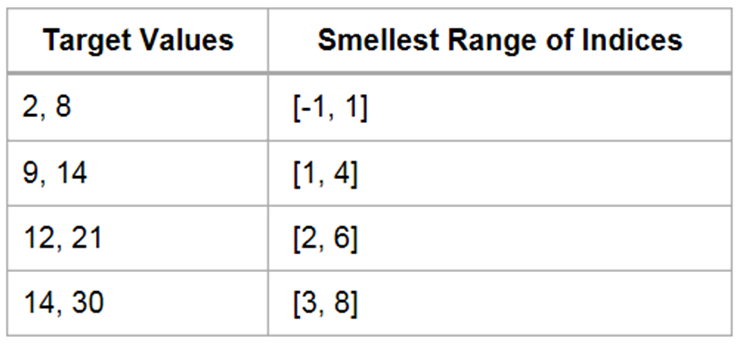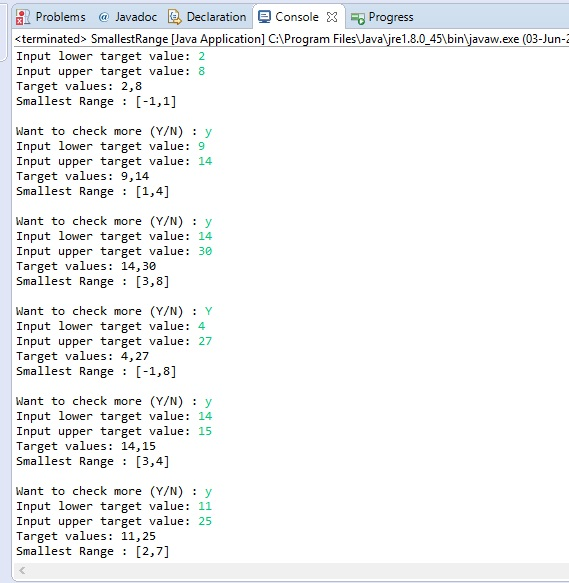# Answered! I appreciate any help with the following Java problem, pleae only use Java format for the program….

I appreciate any help with the following Java problem, pleae only use Java format for the program.

Consider an array data of n numerical values in sorted order and a list of two numerical target values. Your goal is to compute the smallest range of array indices that contains both of the target values. If a target value is smaller than data, the range should start with a -1. If a target value is larger than data[n-1], the range should end with n.

Don't use plagiarized sources. Get Your Custom Essay on
Answered! I appreciate any help with the following Java problem, pleae only use Java format for the program….
GET AN ESSAY WRITTEN FOR YOU FROM AS LOW AS \$13/PAGE

For example, given the arrayThe following table illustrates target values and their corresponding ranges:— Devise and implement an algorithm that solves this problem —

JAVA CODE

package compute.smallestRange;

import java.util.Scanner;

public class SmallestRange {

private static int sIndex;

private static int eIndex;

public static void main(String[] args)

{

int[] array = {5, 8, 10, 13, 15, 20, 22, 26}; // declare your required array here

int lowerTarget;

int upperTarget;

String st;

Scanner input = new Scanner(System.in);

while(true){ // loop to continuously input the target values

System.out.print(“Input lower target value: “);

lowerTarget = Integer.parseInt(input.nextLine());

System.out.print(“Input upper target value: “);

upperTarget = Integer.parseInt(input.nextLine());

ComputeSmallestRange(array, lowerTarget, upperTarget);

System.out.println(“Target values: ” + lowerTarget+”,”+upperTarget);

System.out.println(“Smallest Range : ” + “[“+sIndex+”,”+ eIndex+”]n”);

System.out.print(“Want to check more (Y/N) : “);

st = input.nextLine();

if(st.equals(“Y”) || st.equals(“y”))

continue;

else

System.exit(0);

}

}

/**

* This method will take below arguments as input and calculate the smallest range

* @param array

* @param Lower Target=LT

* @param Upper Target=UT

*/

public static void ComputeSmallestRange(int[] array, int LT, int UT)

{

sIndex = array.length-1; // Initializing the index with last so that if target not found and is greater then

// last element then it simply means that it lies outside of array

eIndex = array.length; // Same with last index

for(int i=0; i<array.length-1; i++) // looping through elements of variable

{

if(i==0)

{

if(array[i]>LT)

sIndex = -1;

if(array[i]>UT)

eIndex = 0;

}

else

{

if(array[i] <= LT && array[i+1]>LT)

{

if(array[i]<=LT)

sIndex = i;

else

sIndex = i+1;

}

if(array[i] <= UT && array[i+1]>UT)

{

if(array[i] == UT)

eIndex = i;

else

eIndex = i+1;

}

}

}

}

}

OUTPUTDIRECTIONS : Open your java ide create a new project with package name compute.smallestRange and within that package create a new class named SmallestRange and then copy-paste the above code into the file and run.